/

### Function Description

Returns an array of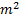elements corresponding to the elements of the matrix,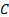, of Spearman rank correlation coefficients for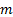series that are provided to the function as an array of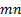elements, where the firstelements correspond to the terms in the first series, the nextelements to the corresponding terms in the second series etc.

Spearman rank correlation coefficients are calculated as: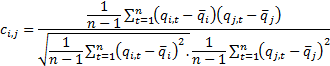where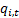are the ranks of the values in the series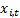, i.e.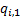is the largest value in the series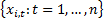,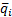is the mean of the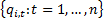etc.

Contents | Prev | Next